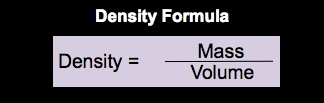Start typing, then use the up and down arrows to select an option from the list.## General Chemistry

Learn the toughest concepts covered in Chemistry with step-by-step video tutorials and practice problems by world-class tutors

1. Intro to General Chemistry

Density represents the mass of an object or compound within a given volume.

Understanding Density
1
concept

## Density1m
Play a video:
so density represents the amount of mass per unit of volume. And here we have our purple box again. Remember, when we have a purple box, that means that is a formula or some type of concept you need to commit to memory. So density is equal to mass over volume. Depending on the phase of matter, the units Ford can be different. So we're gonna say for solids and liquids which are more dense than gasses, solids and liquids have the unit for mass in grams and a unit for volume in middle leaders. Or they have the unit for mass still in grams or the units for volume in Centimeters Cube. Remember when we talked about conversion factors for volume? We said that one middle leader was equal toe one centimeters. Cute. That's why we're allowed to basically swap out MLS here for centimeters cubes here. Now, gasses themselves are much less dense than solids and liquids, so their units for density are a little bit different, their masses still Ingram's. But now, because they're less dense, we wouldn't use milliliters, would use leaders and remember within our conversion factors. We said that one mil on one leader was equal toe one Desa meters cute. So we could say the density of gas is is grams per leader or grams per desa meters cute. So just remember, density itself is just massive volume. Depending on the face of matter that we're dealing with, the units can be slightly different. Now that we've looked at the basic set up of density, let's move on to our example and practice questions.2
example

## Density Example 15m
Play a video: